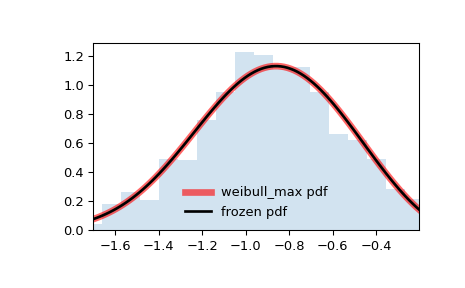# scipy.stats.weibull_max¶

scipy.stats.weibull_max = <scipy.stats._continuous_distns.weibull_max_gen object>[source]

Weibull maximum continuous random variable.

The Weibull Maximum Extreme Value distribution, from extreme value theory (Fisher-Gnedenko theorem), is the limiting distribution of rescaled maximum of iid random variables. This is the distribution of -X if X is from the weibull_min function.

As an instance of the rv_continuous class, weibull_max object inherits from it a collection of generic methods (see below for the full list), and completes them with details specific for this particular distribution.

Notes

The probability density function for weibull_max is:

$f(x, c) = c (-x)^{c-1} \exp(-(-x)^c)$

for $$x < 0$$, $$c > 0$$.

weibull_max takes c as a shape parameter for $$c$$.

The probability density above is defined in the “standardized” form. To shift and/or scale the distribution use the loc and scale parameters. Specifically, weibull_max.pdf(x, c, loc, scale) is identically equivalent to weibull_max.pdf(y, c) / scale with y = (x - loc) / scale. Note that shifting the location of a distribution does not make it a “noncentral” distribution; noncentral generalizations of some distributions are available in separate classes.

References

https://en.wikipedia.org/wiki/Weibull_distribution

https://en.wikipedia.org/wiki/Fisher-Tippett-Gnedenko_theorem

Examples

>>> from scipy.stats import weibull_max
>>> import matplotlib.pyplot as plt
>>> fig, ax = plt.subplots(1, 1)


Calculate the first four moments:

>>> c = 2.87
>>> mean, var, skew, kurt = weibull_max.stats(c, moments='mvsk')


Display the probability density function (pdf):

>>> x = np.linspace(weibull_max.ppf(0.01, c),
...                 weibull_max.ppf(0.99, c), 100)
>>> ax.plot(x, weibull_max.pdf(x, c),
...        'r-', lw=5, alpha=0.6, label='weibull_max pdf')


Alternatively, the distribution object can be called (as a function) to fix the shape, location and scale parameters. This returns a “frozen” RV object holding the given parameters fixed.

Freeze the distribution and display the frozen pdf:

>>> rv = weibull_max(c)
>>> ax.plot(x, rv.pdf(x), 'k-', lw=2, label='frozen pdf')


Check accuracy of cdf and ppf:

>>> vals = weibull_max.ppf([0.001, 0.5, 0.999], c)
>>> np.allclose([0.001, 0.5, 0.999], weibull_max.cdf(vals, c))
True


Generate random numbers:

>>> r = weibull_max.rvs(c, size=1000)


And compare the histogram:

>>> ax.hist(r, density=True, histtype='stepfilled', alpha=0.2)
>>> ax.legend(loc='best', frameon=False)
>>> plt.show()Methods

 rvs(c, loc=0, scale=1, size=1, random_state=None) Random variates. pdf(x, c, loc=0, scale=1) Probability density function. logpdf(x, c, loc=0, scale=1) Log of the probability density function. cdf(x, c, loc=0, scale=1) Cumulative distribution function. logcdf(x, c, loc=0, scale=1) Log of the cumulative distribution function. sf(x, c, loc=0, scale=1) Survival function (also defined as 1 - cdf, but sf is sometimes more accurate). logsf(x, c, loc=0, scale=1) Log of the survival function. ppf(q, c, loc=0, scale=1) Percent point function (inverse of cdf — percentiles). isf(q, c, loc=0, scale=1) Inverse survival function (inverse of sf). moment(n, c, loc=0, scale=1) Non-central moment of order n stats(c, loc=0, scale=1, moments=’mv’) Mean(‘m’), variance(‘v’), skew(‘s’), and/or kurtosis(‘k’). entropy(c, loc=0, scale=1) (Differential) entropy of the RV. fit(data) Parameter estimates for generic data. See scipy.stats.rv_continuous.fit for detailed documentation of the keyword arguments. expect(func, args=(c,), loc=0, scale=1, lb=None, ub=None, conditional=False, **kwds) Expected value of a function (of one argument) with respect to the distribution. median(c, loc=0, scale=1) Median of the distribution. mean(c, loc=0, scale=1) Mean of the distribution. var(c, loc=0, scale=1) Variance of the distribution. std(c, loc=0, scale=1) Standard deviation of the distribution. interval(alpha, c, loc=0, scale=1) Endpoints of the range that contains fraction alpha [0, 1] of the distribution